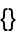#Function Repository Resource:

# QuasiPerfectNumberQ

Test whether an integer is a quasiperfect number

Contributed by: Sander Huisman
 ResourceFunction["QuasiPerfectNumberQ"][n] tests whether n is a quasiperfect number.

## Details

n must be an integer.
The test will be done on Abs[n] if n is negative.
Quasiperfect numbers, if they exist, will be >1035, have at least 7 distinct prime factors and are odd squares.
Quasiperfect returns either True or False.
No quasiperfect number is known.

## Examples

### Basic Examples (2)

Try a number:

 In:=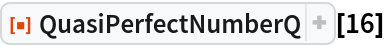Out=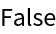Negative numbers are tested as if they are positive:

 In:=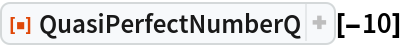Out=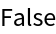### Neat Examples (2)

Try out the numbers 1 through 107:

 In:=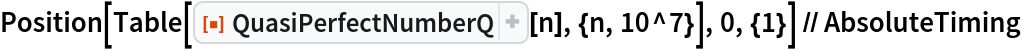Out=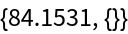Test the first 10,000 odd square numbers after 1036:

 In:=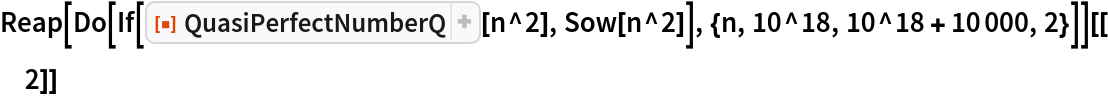Out=